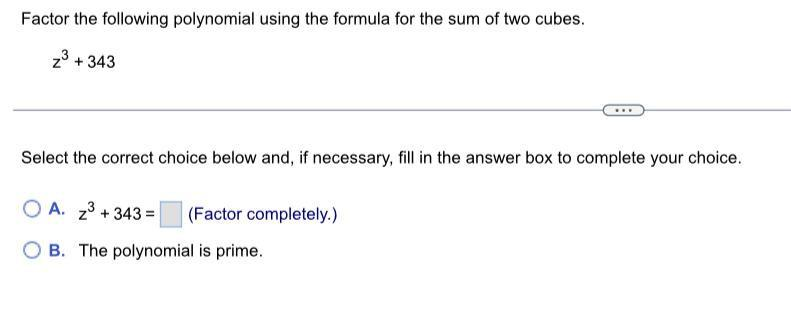Question:

# Factor the following polynomial using the formula for the

Last updated: 8/1/2022Factor the following polynomial using the formula for the sum of two cubes. z³+343 Select the correct choice below and, if necessary, fill in the answer box to complete your choice. A. z³ + 343 = __________(Factor completely.) B. The polynomial is prime.